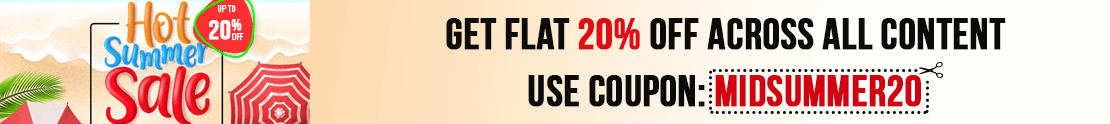# User ForumSubject :IMO    Class : Class 7

Class : Class 1

Subject :IMO    Class : Class 7

Class : Class 1

Subject :IMO    Class : Class 7

Class : Class 1

Subject :IMO    Class : Class 3

Class : Class 3
ans-(A)

## Ans 2:

Class : Class 1
(a) ten and half

Subject :IMO    Class : Class 5

## Ans 1:

Class : Class 8
could you give me the working of the Q

Class : Class 5
1

## Ans 3:

Class : Class 5
Calculate squares of all numbers and then add them up. 7x7=49 2x2=4 1x1=1 49 4 1=54.

Class : Class 5
How?

## Ans 5:

Class : Class 8
1st figure: 22 72 12 = 542nd figure: 42 82 72 = 1293rd figure: ? = 32 92 52 = 115

Class : Class 6

Class : Class 6

Class : Class 6

Class : Class 6

## Ans 10:

Class : Class 6

Subject :IMO    Class : Class 3

## Ans 1:

Class : Class 3
Answer is 64. sides of shape multiply with same. 3*3=9 ,4*4= 16,5*5= 25, 8*8=64

Subject :IMO    Class : Class 7

## Ans 1:

Class : Class 8
The answer is option A ,4 as all squares are rectangle because all sides are parallel

## Ans 2:

Class : Class 8
But 4 and 4 is a square

## Ans 3:

Class : Class 10
The answer is option A because the rectangles formed will be of length 1 and 7, 2 and 6, 3 and 5, 4 and 4. Since the sum of these is 8 and perimeter=2(l+b) thus 2(8) = 16

## Ans 4:

Class : Class 7

Subject :IMO    Class : Class 7

## Ans 1:

Class : Class 9
plz plz plz find the answer and tell me at arijit.01paul@gmail.com with solution

Class : Class 8

## Ans 3:

Class : Class 7
i think the question is wrong ....correct answer is 19x/12

Subject :IMO    Class : Class 1

Class : Class 1
2+2/2 = 2+1 =3.

Class : Class 1
2+2/2 = 2+1 = 3.

Class :
3